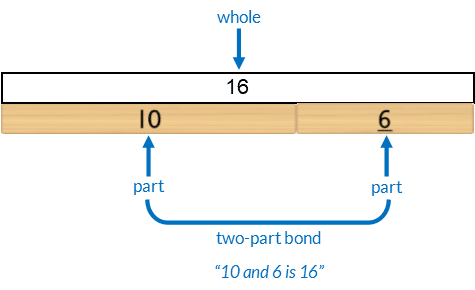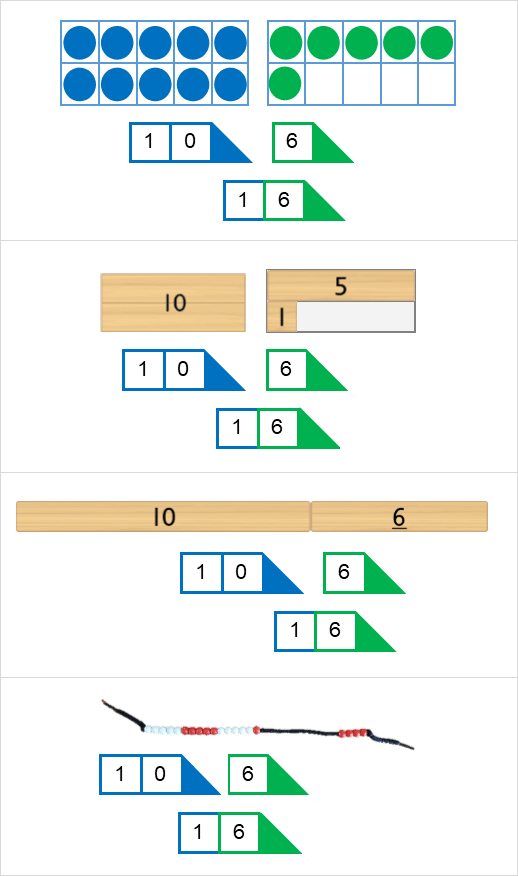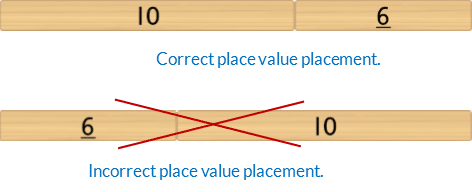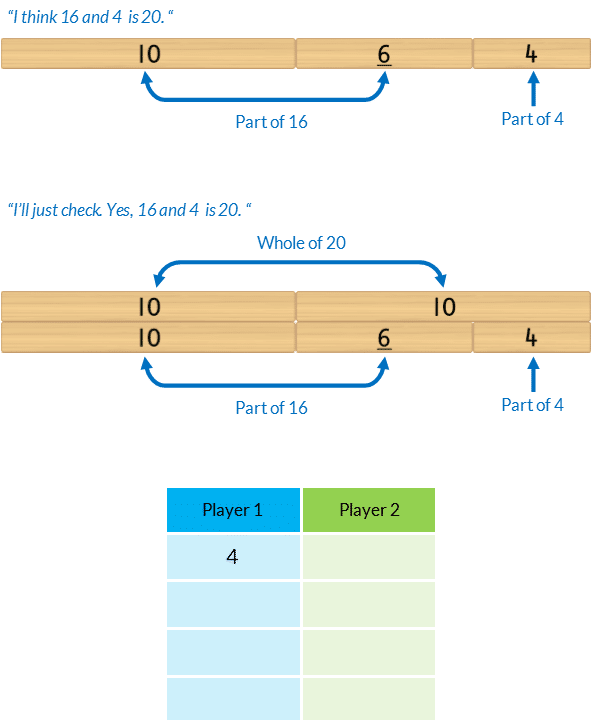## 42) Bonds

Ten Plus Bonds: Multiple Representations### Mathematics

Develop the concept of place value for numbers 11 to 20 representing this partition using:

• ten frames with counters
• empty 10 frame Bond Block
• linear ten Bond Block

Begin to relate the place value partition to addition of ten and a single-digit part. For example, 16 is 10 and 6.

### Language

• ten plus bond: a bond of ten and one other part makes the whole.
• equals as “is”• A number is made up of digits in the same way a word is made up of letters. The number 16 is made up of the digits one and six.
• Place value: The place of a digit in a number determines the digit’s value (worth).
• In the number 16 the digit one is in the tens place so is worth one ten, ten.
• The digit six is in the ones place so is worth six ones, six.## Differentiation

### A little easier

##### Use place value arrow cards

Click to download Place Value Arrow Cards. Use these in conjunction with manipulatives on the activity board to help students make connections between the manipulatives and written value of each digit.When filling tens frames fill the top row first, filling from the left. Discourage students from counting. Relate each row to one hand of 5 fingers.

When placing blocks use place value. Place the ten on the left and the ones block on the right as per the written number.### A little harder

##### How many more to 20?

Play Activity 42 Ten Plus Bonds: Bonds Multiple Representations as instructed except change the scoring. The player’s score becomes how many more is needed to make 20. At the end of several rounds students add their scores. The player with the lowest score wins.

For example,  for a spin of 16 the player would score 4.### Progression

In the next activity students develop fluency with numbers up to 29 that have been partitioned using place value.

• In Activity 42 students were given the number (for example, 16) and had to partition this using place value (into 10 and 6).
• They do the reverse in the next activity. They are given the place value partition (for example, 1 ten and 6 ones) and have to write the number (16).

Go to

##### Activity 43

Ten Plus Bonds: Bonds, Place Value Partitioning